# Pump Head Calculation Example – A Guide in Engineering

The head losses in the pipeline consist of linear head losses and local losses on the elements of pipeline systems. Linear head losses are pressure losses due to friction in the pipe; they depend on the flow regime, temperature, and water flow rate, as well as on the wall roughness and pipe diameter. To go deeper in the issue, you should read through the following pump head calculation example.

The sample you will find below calculates the head losses in the pump and determines the rate of flow of water in it. Our blog also includes examples of assignments for other disciplines. All samples are done by experts in the field. You can be sure that the examples are correct, and you can use them to work on your own assignments. So, if you are a student who studies engineering, you will find this pump head calculation example very helpful.

Task: in this guide we will perform m head loss calculations, solve flow rate problems, generate system curves, and find the design point for a system and pump for pipe flow analysis.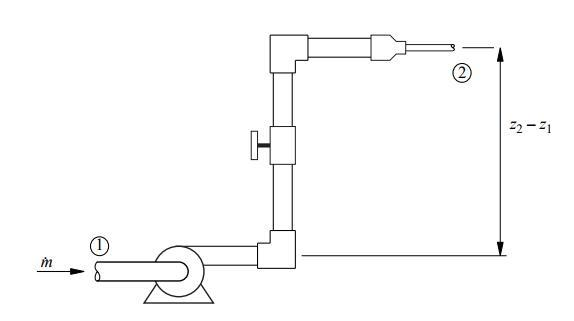Solution:

(Part 1)

Governing Equations

The figure above shows a single pipe flow system. For steady flow between station 1 and station 2, here is the mass conversion: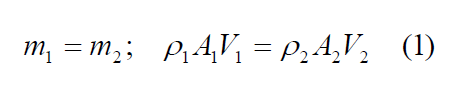Where ˙m is the mass flow rate, ρ is the fluid density, A is the cross-section area, and V is the average velocity. In most applications the flow is incompressible, so ρ1 = ρ2 and Equation (1) simplifies to: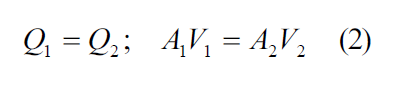where Q is the volumetric flow rate.

Energy conservation between two stations: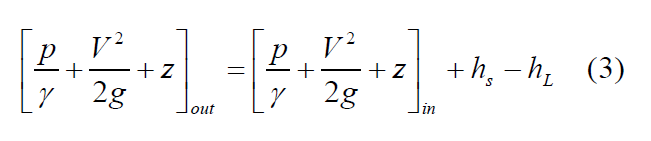Where the subscript “out” indicates the downstream station, and “in” indicates the upstream station, γ = ρg is the specific weight of the fluid, hs is the shaft work done on the fluid, and hL is the head loss due to friction.

To convert hs and hL to units of power we use: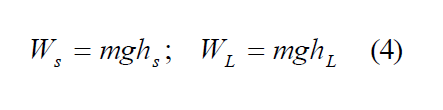The head loss due to friction is given by the Darcy-Weisbach equation: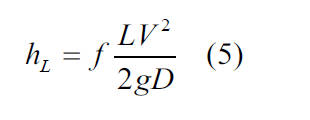Where f is the Darcy Friction Factor. For fully-developed, incompressible laminar flow in a round pipe, the equation is as follows: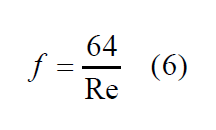The friction factor for turbulent flow in smooth and rough pipes is correlated with the

Colebrook equation: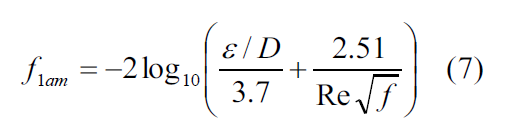Where ε is the roughness of the pipe wall, and Re is the Reynolds number: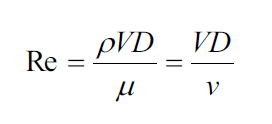If the pipe is not round, the same formulas may be applied if the hydraulic diameter, Dh, is substituted for D in the definition of Re, and in the ε/D term in the Colebrook equation. For a pipe with any cross-section area A and perimeter P, the hydraulic diameter is: